﻿ 基于边缘约束的自适应引导滤波立体匹配算法
«上一篇文章快速检索 高级检索

 应用科技2020, Vol. 47Issue (4): 47-53  DOI: 10.11991/yykj.2020010170

### 引用本文CHEN Liwei, LI Shankun. An adaptive guided filtering stereo matching algorithm based on edge constraints[J]. Applied Science and Technology, 2020, 47(4): 47-53. DOI: 10.11991/yykj.202001017.### 文章历史

An adaptive guided filtering stereo matching algorithm based on edge constraints
CHEN Liwei, LI ShankunCollege of Information and Communication Engineering, Harbin Engineering University, Harbin 150001, China
Abstract: In order to solve the problem of discontinuity in the boundary region of depth map in local stereo matching algorithm, an adaptive guided filtering stereo matching algorithm is proposed based on edge constraint. Firstly, the gradient value and color information are combined to calculate the matching cost; further, based on the local features of the image edge, an adaptive cross region is constructed for the pixels of the image based on the color threshold and boundary conditions, and in the meanwhile, the guided filtering cost aggregation of adaptive window is conducted. Finally, the disparity is calculated by using the winner-takes-all strategy, and the disparity map is refined. Experimental results show that the depth map generated by this algorithm can better retain the detail features, and the mismatch phenomenon in the boundary texture area is significantly improved. This algorithm can effectively reduce the mismatch rate, and the mismatch rate of it in Middlebury dataset is only 5.22%.
Keywords: stereo matching    guided filtering    cost calculation    cross region    cost aggregation    disparity post-processing    Canny algorithm    weighted median filtering

1 局部立体匹配算法框架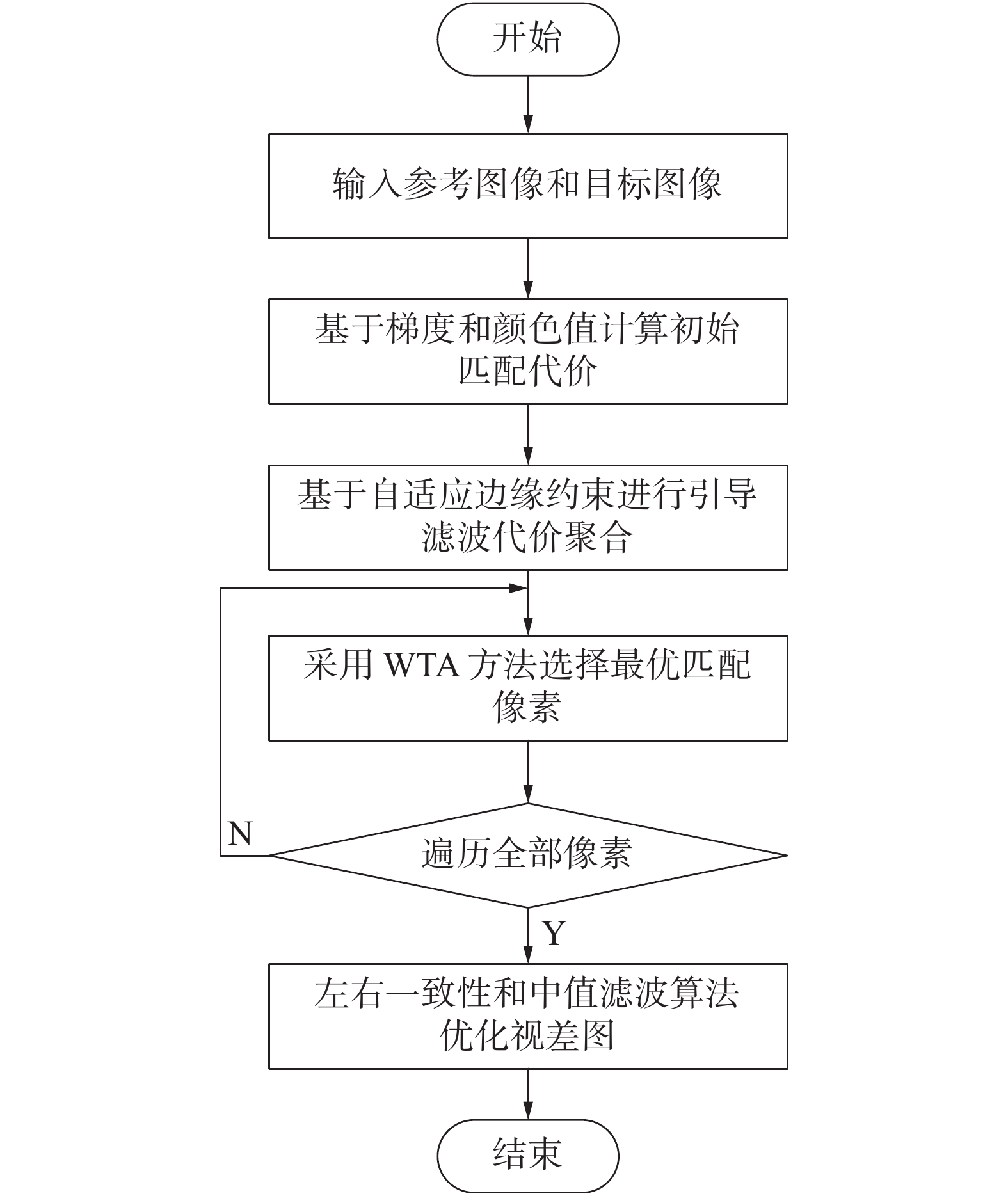Download: 图 1 基于边缘约束的自适应引导滤波立体匹配算法流程

2 本文立体匹配算法 2.1 匹配代价计算

 $\begin{array}{*{20}{c}} {C(i,d) = \alpha \min \left( {\left\| {{I_l}(i) - {I_r}\left( {{i_d}} \right),{T_1}} \right\|} \right)}+\\ {\left. { (1 - \alpha ) \min \left( {\left\| {{\nabla _x}{I_l}(i) - {\nabla _x}{I_r}\left( {{i_d}} \right),{T_2}} \right\|} \right)} \right)} \end{array}$

2.2 代价聚合 2.2.1 自适应区域构建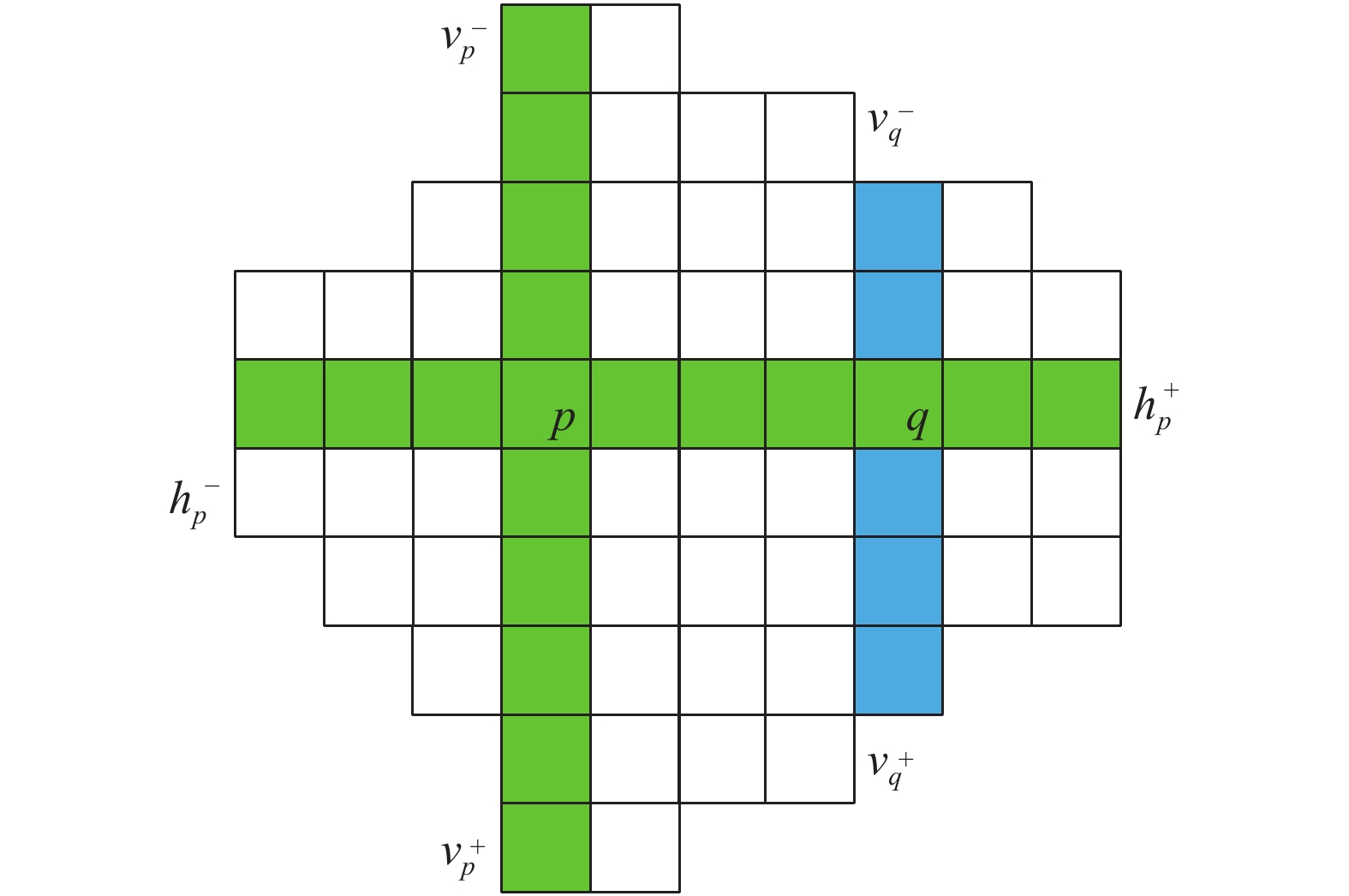Download: 图 2 十字交叉区域构建
 $\delta ({p_1},{p_2}) = \left\{ {\begin{array}{*{20}{l}} {1,}\;\;{\left( {{{\max }_{i = R,G,B}}\left| {{I_i}({P_1}) - {I_i}(P)} \right|} \right) \leqslant \tau } \\ {0,\;\;{\text{其他}}} \end{array}} \right.$ (1)
 ${r^*} = {\max\limits_{r \in [1,L]}}\left( {1,r\prod\limits_{i \in [1,r]} \sigma \left( {p,{p_i}} \right)} \right)$ (2)

 $V(p) = \{ (x,y)|x \in [{x_p} - v_p^ - ,{x_p} + v_p^ + ],y = {y_p}\}$
 $U(P) = \mathop \cup \limits_{q \in V(p)} H(q)$
2.2.2 自适应引导图滤波

 ${C^\prime }(p,d) = \sum\limits_q {{W_{p,q}}} (I)C(q,d)$

 ${Q_i} = {a_k}{I_i} + {b_k},\forall i \in {w_k}$

 $E\left( {{a_k},{b_k}} \right) = \sum\limits_{i \in {w_k}} {\left( {{{\left( {{a_k}{I_i} + {b_k} - {p_i}} \right)}^2} + \varepsilon a_k^2} \right)}$
 ${a_k} = \frac{1}{{|{w_k}|}}\frac{{\displaystyle\sum\nolimits_{i \in {w_k}} {{I_i}{p_i}} - {\mu _k}{{\bar p}_k}}}{{\sigma _k^2 + \varepsilon }}$
 ${b_k} = {\bar p_k} - {a_k}{\mu _k}$

 ${W_{p,q}} = \frac{1}{{{{\left| w \right|}^2}}}\sum\limits_{k:(p,q) \in {w_k}} {\left( {1 + \frac{{({I_p} - {\mu _k})({I_q} - {\mu _k})}}{{\sigma _k^2 + \varepsilon }}} \right)}$ (3)

 ${W_{p,q}} = \frac{1}{{\left| {{w_q}} \right|}}\sum\limits_{k \in {w_p}} {\left( {\frac{1}{{\left| {{w_k}} \right|}}\sum\limits_{q \in {w_k}} {\left( {1 + \frac{{({I_p} - {\mu _k})({I_q} - {\mu _k})}}{{\sigma _k^2 + \varepsilon }}} \right)} } \right)}$

2.2.3 基于边缘约束的自适应引导滤波

 $\tau ({l_{pq}}) = - {\tau _{\max }}/{L_{\max }} \times {l_{pq}} + {\tau _{\max }}$
 ${D_s}(p,q) < {L_{\max }},{D_c}(p,q) < \tau \left( {{l_{pq}}} \right)$
 ${D_s}(p,q) = \left\{ {\begin{array}{*{20}{l}} {\min (r,{l_{pq}}),{{I}}(q) = 1} \\ {\max (r,{l_{pq}}),{\text{其他}}} \end{array}} \right.$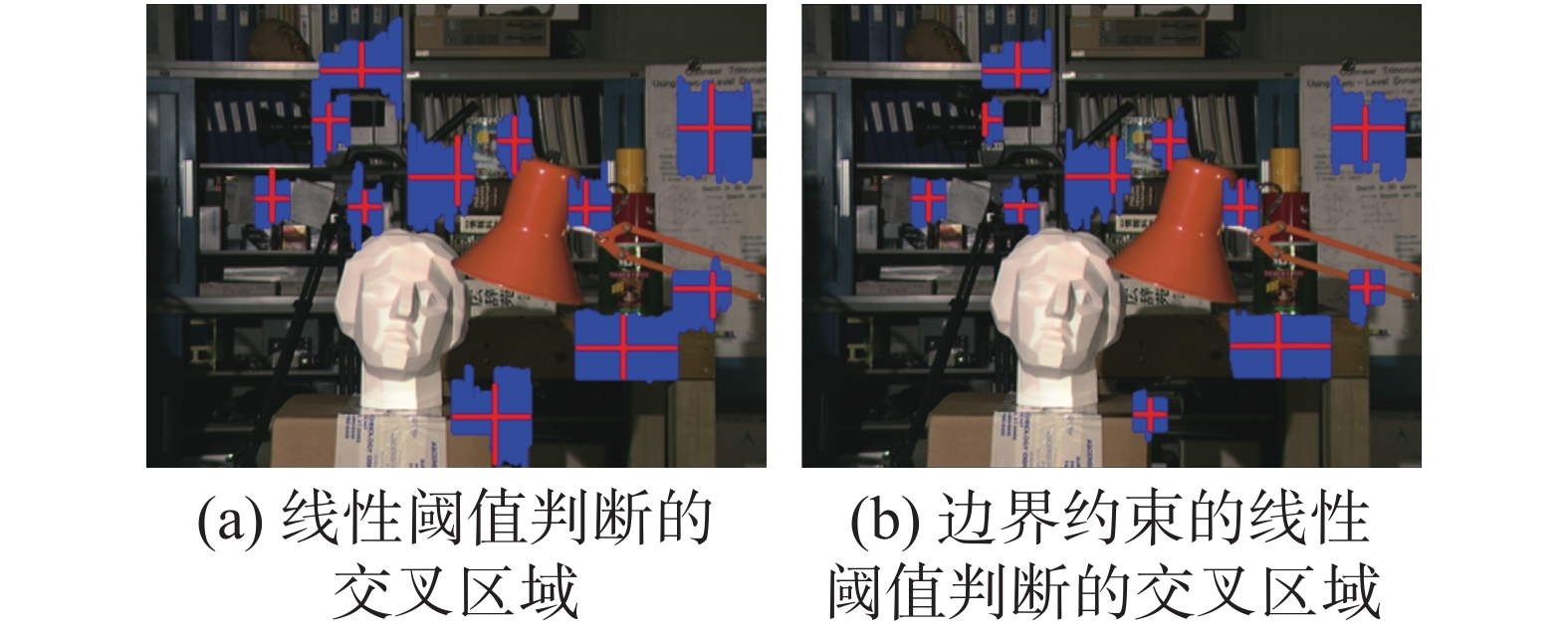Download: 图 3 不同判决条件所构成的交叉区域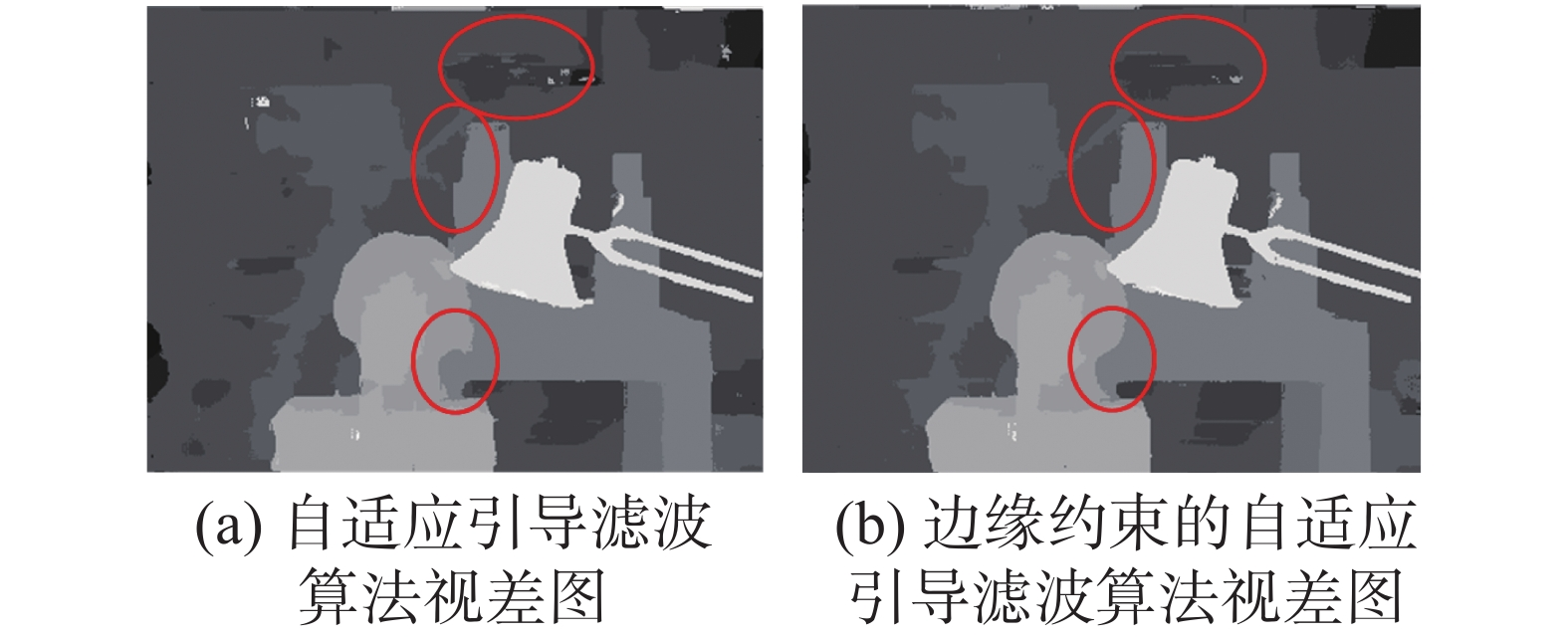Download: 图 4 两种引导滤波算法处理后的视差
2.3 视差计算与精细化后处理

 ${d_p} = \arg \mathop {\min }\limits_{d \in D} [\widehat C(p,d)]$

 $|{d_L}(i) - {d_R}[{\rm{i}} - {d_L}(i)]| < {\delta _0}$

3 实验验证表 1 实验参数设置
3.1 代价聚合算法验证Download: 图 5 引导滤波算法与本文算法对比表 2 不同代价聚合算法误匹配率
3.2 算法有效性验证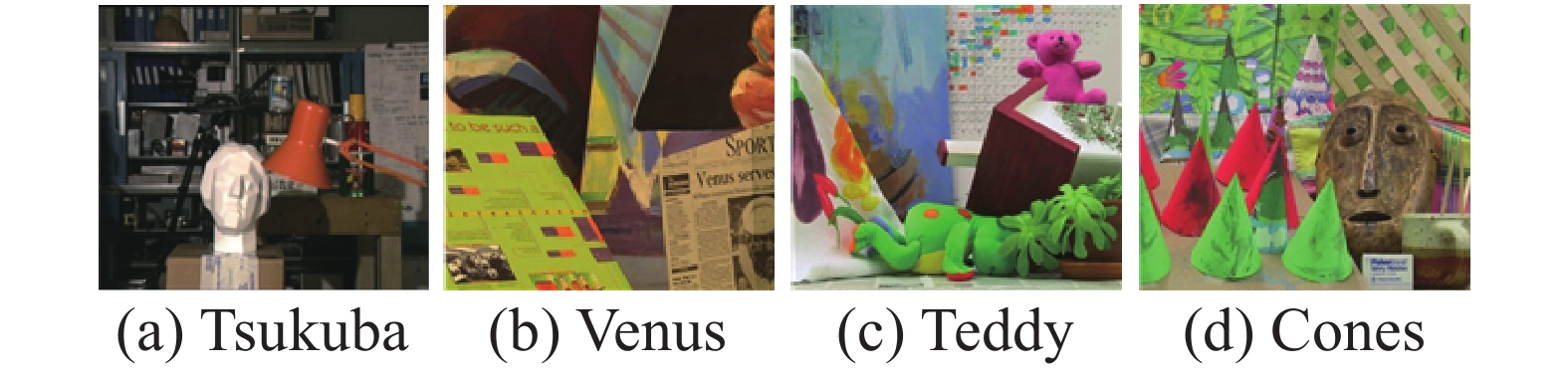Download: 图 6 Middlebury数据集图像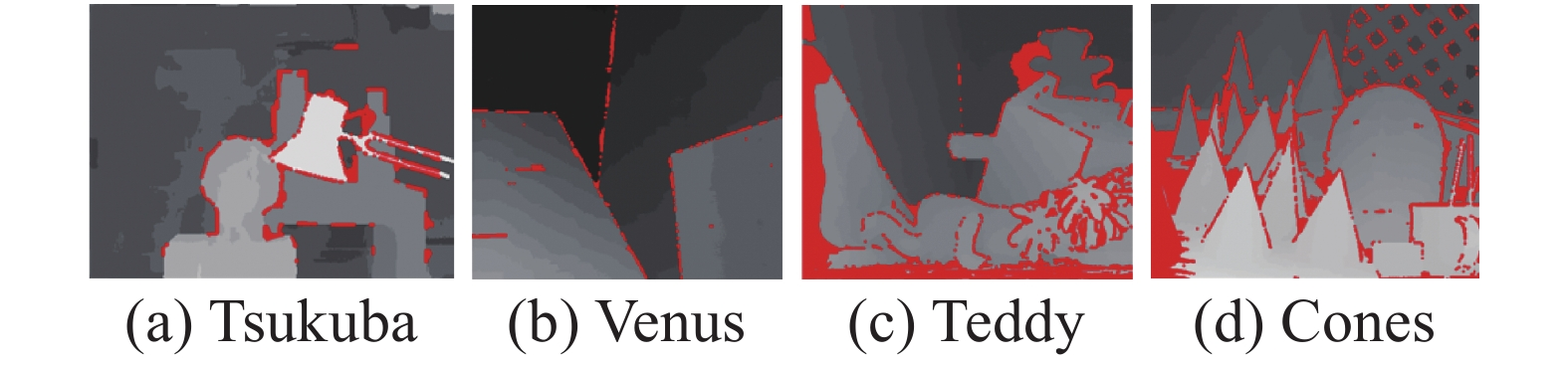Download: 图 7 CostFilter算法误匹配视差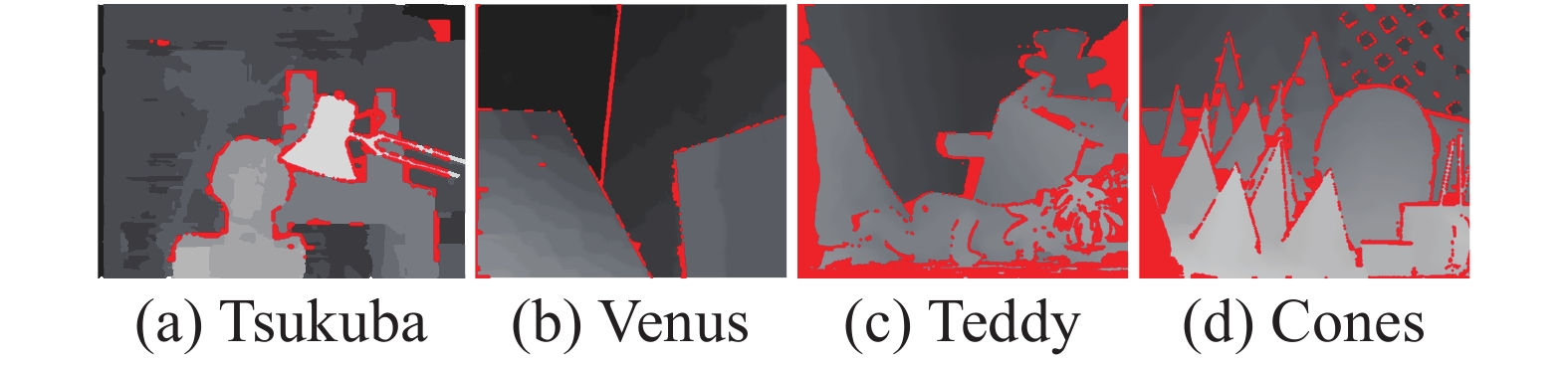Download: 图 8 SMPF算法误匹配视差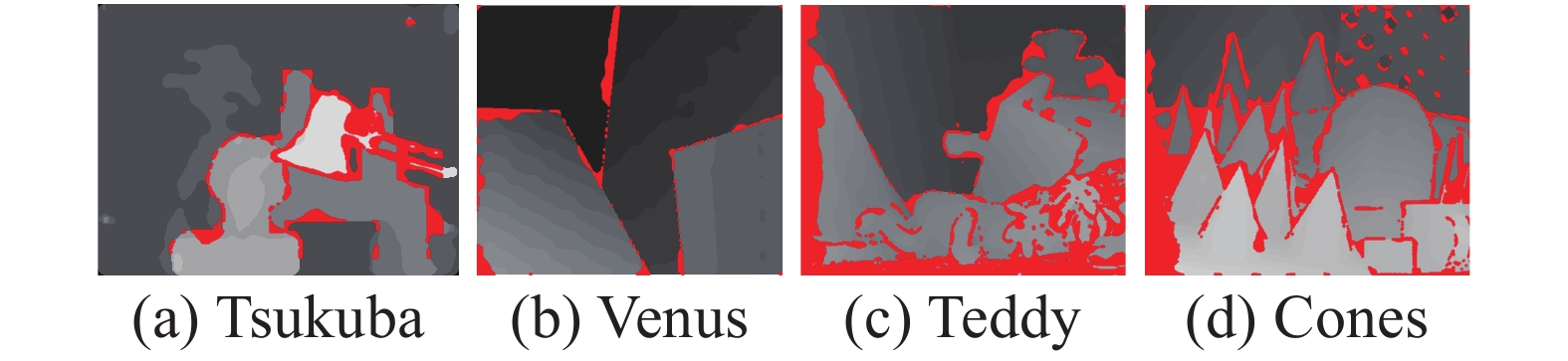Download: 图 9 LCVB-DEM算法误匹配视差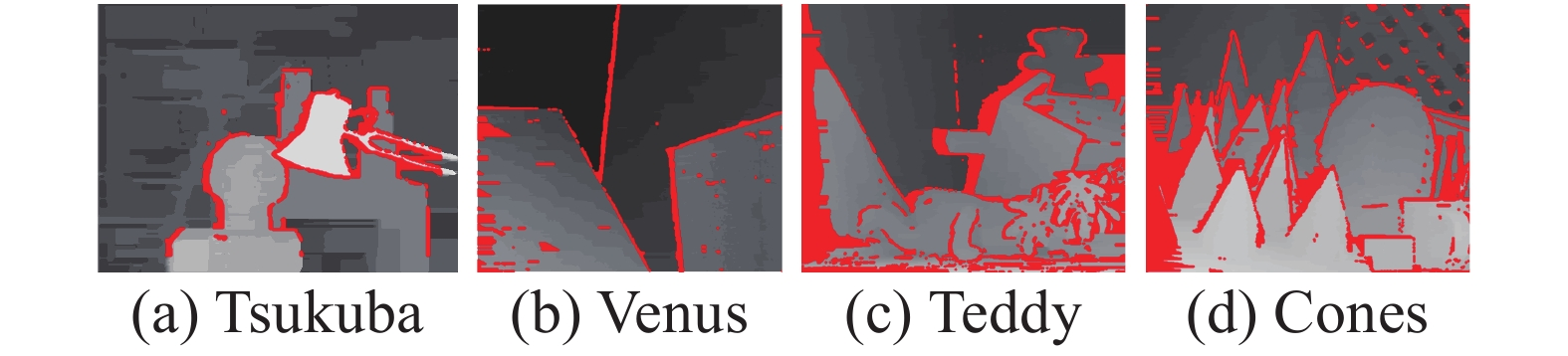Download: 图 10 AdaptAggrDP算法误匹配视差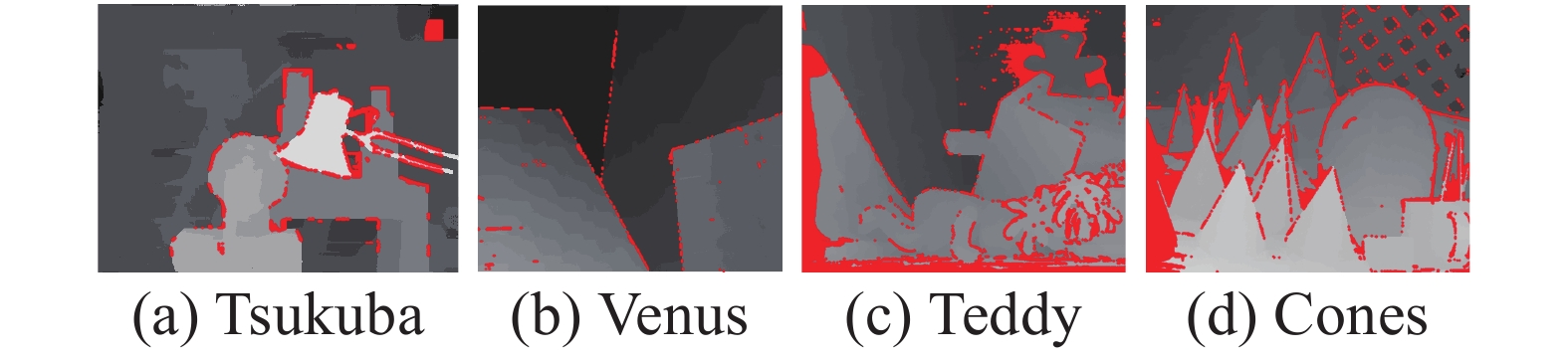Download: 图 11 本文算法误匹配视差表 3 立体匹配算法误匹配率比较

4 结论

1)与固定窗口大小的引导滤波算法相比，本文的自适应引导滤波算法可以根据各个像素点所构建的交叉区域进行滤波操作，充分利用各个像素点的差异性，并且考虑边缘点的特殊部分，使得本文算法在最终视差图中能够较好地保留边界结果。

2)自适应引导滤波由于在代价聚合过程中需要计算每个像素点的交叉区域，所以在算法的整体运算时间上会有所增加，要使立体匹配算法能够真正满足实时性的要求，可以考虑使用并行运算实现加速。

  BLEYER M, BREITENEDER C.Stereo matching state-of-the-art and research challenges [M]. London,Springer, 2013: 143-79. (0)  SCHARSTEIN D, SZELISKI R. Stereo matching with non-linear diffusion; proceedings of the computer vision and pattern recognition[J]. International journal of computer vision, 1998, 28(2): 155-174. (0)  SUN J, SHUM H Y, ZHENG N. Stereo matching using belief propagation[J]. IEEE transactions on pattern analysis and machine intelligence, 2003, 25(7): 787-800. (0)  KOLMOGOROV V, ZABIH R. Computing visual correspondence with occlusions using graph cuts[C]//Proceedings Eighth IEEE International Conference on Computer Vision. Barcelona, Spain, 2001, 2: 508-515. (0)  VAN MEERBERGEN G, VERGAUWEN M, POLLEFEYS M, et al. A hierarchical symmetric stereo algorithm using dynamic programming[J]. International journal of computer vision, 2002, 47(1-3): 275-85. (0)  ZHANG K, LU J, LAFRUIT G. Cross-based local stereo matching using orthogonal integral images[J]. IEEE transactions on circuits and systems for video technology, 2009, 19(7): 1073-1079. DOI:10.1109/TCSVT.2009.2020478 (0)  MEI X, SUN X, ZHOU M, et al. On building an accurate stereo matching system on graphics hardware[C]// Proceedings of 2011 IEEE International Conference on Computer Vision Workshops . Barcelona, Spain, 2011: 467-474. (0)  HE K, SUN J, TANG X. Guided image filtering[J]. IEEE transactions on pattern analysis and machine intelligence, 2012, 35(6): 1397-409. (0)  HOSNI A, RHEMANN C, BLEYER M, et al. Fast cost-volume filtering for visual correspondence and beyond[J]. IEEE transactions on pattern analysis and machine intelligence, 2012, 35(2): 504-11. (0)  闫利, 王芮, 刘华, 等. 基于改进代价计算和自适应引导滤波的立体匹配[J]. 光学学报, 2008, 38(11): 257-67. (0)  HE K, GE Y, ZHEN R, et al. Fast adaptive support-weight stereo matching algorithm[J]. Transactions of Tianjin University, 2017, 23(3): 295-300. DOI:10.1007/s12209-017-0034-5 (0)  葛云峰. 自适应权重立体匹配算法研究 [D].天津: 天津大学, 2017. (0)  王志, 朱世强, 卜琰. 改进导向滤波器立体匹配算法[J]. 浙江大学学报(工学版), 2016, 50(12): 2262-2269. (0)  黄学然. 基于双目立体视觉的三维重建技术研究 [D].西安: 西安电子科技大学, 2018. (0)  程德强, 庄焕东, 于文洁, 等. 基于边缘加权的跨尺度局部立体匹配算法[J]. 激光与光电子学进展, 2019, 56(21): 156-64. (0)  CANNY J. A computational approach to edge detection[J]. IEEE transactions on pattern analysis and machine intelligence, 1986(6): 679-698. (0)  SCHARSTEIN D, PAL C. Learning conditional random fields for stereo[C]// Proceedings of 2007 IEEE Conference on Computer Vision and Pattern Recognition. Minneapolis, Minnesota, 2007: 1-8. (0)  MARTINS J A, RODRIGUES J M, DU BUF H. Luminance, colour, viewpoint and border enhanced disparity energy model[J]. PloS one, 2015, 10(6): e0129908. DOI:10.1371/journal.pone.0129908 (0)  PLOUMPIS S, AMANATIADIS A, GASTERATOS A. A stereo matching approach based on particle filters and scattered control landmarks[J]. Image and vision computing, 2015, 38: 13-23. DOI:10.1016/j.imavis.2015.04.001 (0)  WANG L, YANG R, GONG M, et al. Real-time stereo using approximated joint bilateral filtering and dynamic programming[J]. Journal of real-time image processing, 2014, 9(3): 447-461. DOI:10.1007/s11554-012-0275-4 (0)